# Requests & Ideas - page 11449

Fellow members, I am looking for advice.

Pease forgive me if I enter this in the wrong forum.

I have attached a picture of a chart of the AUSUSD pair, but it doesn't matter the pair. What I am looking for is a way to ignore the flat market. The picture shows a 5 minute chart, with OMA 1000 length, 100, 150, 300 etc. It shows the market going side ways.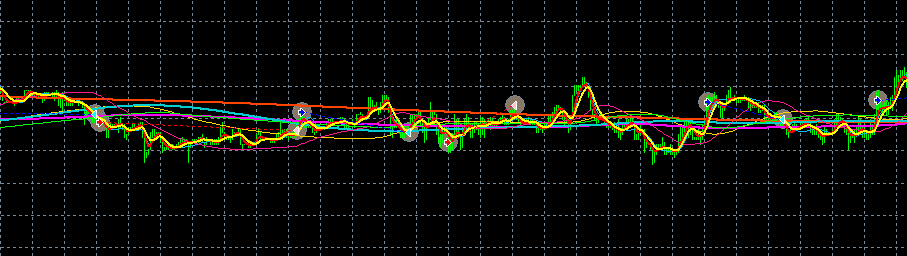What king of indicator can someone suggest( and how to use it) to ignore this type of market condition?

gorick

Files:118

PAL Divergence

mrtools (or other):

I've noticed you're very good at adding divergence to indicators. Might I ask that for the attached? Thanks.

palnd_v1.58.mq4

Files:9493

mrtools (or other):

I've noticed you're very good at adding divergence to indicators. Might I ask that for the attached? Thanks.

palnd_v1.58.mq4

Here you go!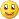Files: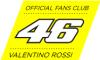158

How to "Weight" an indicator value along all time frames...

Hi everybody,

I've not a math PhD so I'm looking for help of somebody that "chew" math like eating popcorn in front of the TV...I'm trying to develop a sort of "directional trend strength analyzer" that monitor all time frames from M1 to W1 with 3 indicators; I can assign to each indicator a "trend score (TS)" value from -n to +n (related to the reading of the indicator itself); now I'm able to establish the "single time frame trend score (STFTS)", for example simply adding the trend score values of each indicator: STFTS_M1 = TS1_M1 + TS2_M1 + TS3_M1; STFTS_M5 = TS1_M5 + TS2_M5 + TS3_M5 ....... STFTS_W1 = TS1_W1 + TS2_W1 + TS3_W1.

My real problem is to find a correct method (aka formula or algorithm...) to give a proper "weight" to each STFTS, to calculate the "total trend score (TTS)", related to the time frame of the chart where the indicator will be applied.

In detail: I could (in example) just sum all the STFTS, then divide by the number of time frames and I'll get a "simple average" (TTS = (STFTS_M1 + STFTS_M5 +........ STFTS_W1)/8) of the TTS.

Well, this data is not really helpful 'cause, in this way, each time frame contribute to the overall, with the same "power" or "weight" so to speak... therefore if you apply this kind of calculation you'll have the same value for the TTS no matter which time frame of the chart the indicator is applied to; my goal is to find a way to give a "weighted" power for each STFTS related to the time frame of the chart where the analyzer is applyed to.

So if I do apply the analyzer to a M15 chart for example, I want to calculate the TTS giving more weight to the neighbor time frames and less to the far away; something like: TTS_M15 = (STFTS_M1(80%) + STFTS_M5(90%) STFTS_M15(100%) + STFTS_M30(80%) + STFTS_H1(60%) + STFTS_H4(40%) + STFTS_D1(20%) + STFTS_W1(10%))/8 ; or if I apply the analyzer to a 4H chart something like: TTS_H4 = (STFTS_M1(5%) + STFTS_M5(10%) STFTS_M15(15%) + STFTS_M30(20%) + STFTS_H1(40%) + STFTS_H4(100%) + STFTS_D1(70%) + STFTS_W1(30%))/8; trying to get a better "responsiveness" of the indicator itself; sort of adaptivity of the analyzer (as paragon like an exponential moving average that give more "importance" to the recent values respect to the oldest, but the exponential calculation cannot be applied in this case...).

Somebody out there might be so kind to help me to find out how to properly calculate the percentage that has to be applied to the "single time frame trend score"????

Thanks to anybody that did spent 2 precious minutes of his own life reading this delirant postBest Regards

brax2078

Brownian motion

brax64:
Hi everybody,

I've not a math PhD so I'm looking for help of somebody that "chew" math like eating popcorn in front of the TV...

I'm trying to develop a sort of "directional trend strength analyzer" that monitor all time frames from M1 to W1 with 3 indicators; I can assign to each indicator a "trend score (TS)" value from -n to +n (related to the reading of the indicator itself); now I'm able to establish the "single time frame trend score (STFTS)", for example simply adding the trend score values of each indicator: STFTS_M1 = TS1_M1 + TS2_M1 + TS3_M1; STFTS_M5 = TS1_M5 + TS2_M5 + TS3_M5 ....... STFTS_W1 = TS1_W1 + TS2_W1 + TS3_W1.

My real problem is to find a correct method (aka formula or algorithm...) to give a proper "weight" to each STFTS, to calculate the "total trend score (TTS)", related to the time frame of the chart where the indicator will be applied.

In detail: I could (in example) just sum all the STFTS, then divide by the number of time frames and I'll get a "simple average" (TTS = (STFTS_M1 + STFTS_M5 +........ STFTS_W1)/8) of the TTS.

Well, this data is not really helpful 'cause, in this way, each time frame contribute to the overall, with the same "power" or "weight" so to speak... therefore if you apply this kind of calculation you'll have the same value for the TTS no matter which time frame of the chart the indicator is applied to; my goal is to find a way to give a "weighted" power for each STFTS related to the time frame of the chart where the analyzer is applyed to.

So if I do apply the analyzer to a M15 chart for example, I want to calculate the TTS giving more weight to the neighbor time frames and less to the far away; something like: TTS_M15 = (STFTS_M1(80%) + STFTS_M5(90%) STFTS_M15(100%) + STFTS_M30(80%) + STFTS_H1(60%) + STFTS_H4(40%) + STFTS_D1(20%) + STFTS_W1(10%))/8 ; or if I apply the analyzer to a 4H chart something like: TTS_H4 = (STFTS_M1(5%) + STFTS_M5(10%) STFTS_M15(15%) + STFTS_M30(20%) + STFTS_H1(40%) + STFTS_H4(100%) + STFTS_D1(70%) + STFTS_W1(30%))/8; trying to get a better "responsiveness" of the indicator itself; sort of adaptivity of the analyzer (as paragon like an exponential moving average that give more "importance" to the recent values respect to the oldest, but the exponential calculation cannot be applied in this case...).

Somebody out there might be so kind to help me to find out how to properly calculate the percentage that has to be applied to the "single time frame trend score"????

Thanks to anybody that did spent 2 precious minutes of his own life reading this delirant post

Best Regards

brax

Brax,

No need to chew math like eating popcorn in front of a tv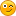...Yours is not a math problem but a conceptual problem.

In practical terms,you can use the ATR relative ratios.

During the last trading hour,ATR(1) for H1,let´s say is 50 pips...you can be sure that ATR(4) for M15 has been 50/square root of 4=25 ....So during THAT hour you shoud have weighted H1 input with double value than M15 input,and,you know?Thanks to brownian motion,no matter what atr you have the next hour,the ratio between H1 and m15 will always be 2(+- rounding).

The ratios would go like this(you can adjust for the exact number of hours,minutes,etc,depending on Sunday trading or not,etc)

W1=X

D1=X/SQRT5= X/2.236...0.4472X

H4=X/SQRT30=X/5.477...0.1826X

H1=X/SQRT180=X/13.416....0.0745X

M30=X/SQRT360=X/18.974...0.0527X

M15=X/SQRT720=X/26.833....0.0373X

M5=X/SQRT2160=X/46.476....0.0215X

M1=X/SQRT10800=X/103.923...0.0096X

Where X=ATR(1) of W1,but you don´t need it,you already have the relative timeframe weights.

Suggestion:I would use 2 composites,one for trend(W1+D1+H4) and the other for timing(H1 to M1) in trend´s direction,2 reasons for this:1-the small relative weight of H1 and below on the total...2-Trader´s behaviour seems to be scinded between those that use H4 and higher(or D1 and Higher) , those that just look to H1 and below and thos ethat us ethe higher tfs for trend and the lower tfs for timing,which is what I suggestRegards

S145

TVI histo

Hello I've seen an TVI volume indy in elite section with a line in the window this one here I found is a non repainting histo version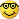perhaps I've missed something in my search here but if there isn't one that already exists can someone direct me to a mtf,interpolate,adaptive lookback,divergence version,basically is there a bells and whistles version for tvi histo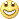,if not please address this,thanks pipsqueaks!Files:
tvi_v2.mq4 9 kb158

Hi Simba,

really thank for your time, your explanation make lots of sense; I'll try to apply the concept and if I'll come up with something interesting I'll post it...

Curiosity; as far as you know there is already out there some indicators that's using a similar approach?

Thank again and

Best Regards

brax

SIMBA:
Brax,

No need to chew math like eating popcorn in front of a tv...Yours is not a math problem but a conceptual problem.

......

......

S163389

Blau tick volume indicator ...

Here is a multi time frame version for nowIt is not the one you posted, but I think it will do (values are the same as the one you posted, since the parameters are set to be the same) One observation though. It is not a tick volume indicator but a signal line of an Ergodic tvi indicator (tvi itself lacks the final 5 period smoothing) Also, original Blau ergodic tvi is calculated as 32,5,5 periods, but I kept the default parameters from the one you posted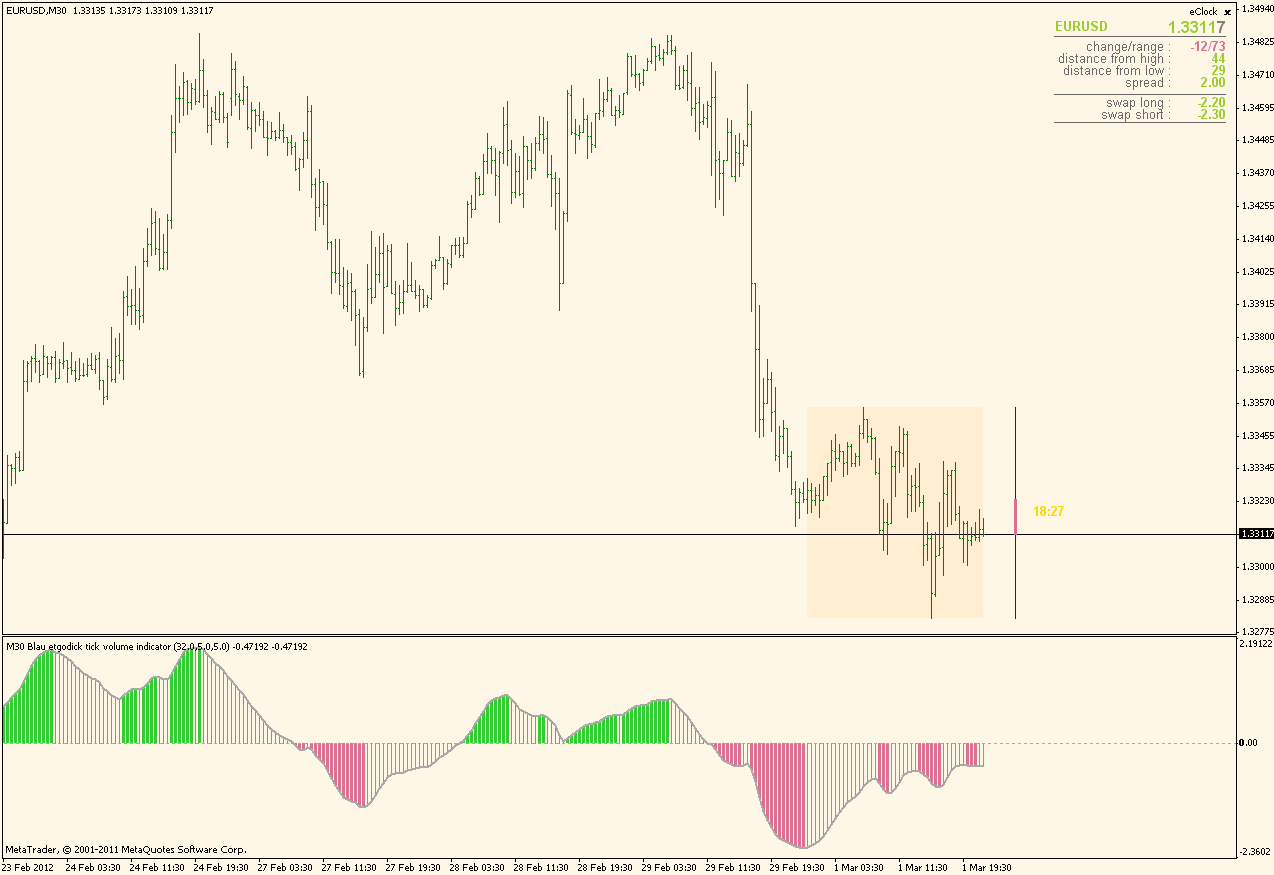Maxwell10:
Hello I've seen an TVI volume indy in elite section with a line in the window this one here I found is a non repainting histo versionperhaps I've missed something in my search here but if there isn't one that already exists can someone direct me to a mtf,interpolate,adaptive lookback,divergence version,basically is there a bells and whistles version for tvi histo,if not please address this,thanks pipsqueaks!Files:
bau_tvi.gif 26 kb145

You Da Man!

thanks this will do, Much love and respect mladen!2078

brax64:
Hi Simba,

really thank for your time, your explanation make lots of sense; I'll try to apply the concept and if I'll come up with something interesting I'll post it...

Curiosity; as far as you know there is already out there some indicators that's using a similar approach?

Thank again and

Best Regards

brax

Brax,

Not that I know.

Please notice that while the weight is biased,as it should, towards the higher tfs,the concept may allow enough responsiveness provided by the lower tfs.

For example,to follow with the simple example for aggregation of H1 and M15 during 1 hour the H1 will be weighted double than the m15,but there will be 4 counts for the m15 value.

Presume a downtrend and your n=10 so both m15 and h1 are at -10 and your composite signals -30...then a reversal starts,at 00:15,H1 will still signal -10,while M15 signals +5(for example),so your composite signals -15,then at 00:30 m15 signals +10,so,now you can do 2 things:either design your composite to signal -10(10+2*-10) or to "accumulate" the m15 signals so that the total for M15-during that hour is now+15,and in this case the composite should signal 15+2*-10=-5.....

I don´t know which of the 2 alternative would be better,but it will be very interesting to test both of them.Of course,if you use all timeframes between h1 and m15 there is no need to accumulate,in the example above at 00:30 you would have gotten-for example- an m30 +5 so that you have H1=2*-10=-20...M15=+10...M30=1.4142*5=7(rounded)...and the composite would be at -3...I presume that using all the tfs between A and B implies there is no need to accumulate.

S